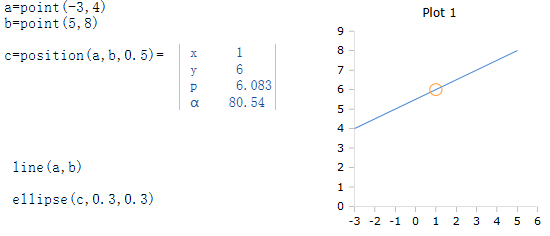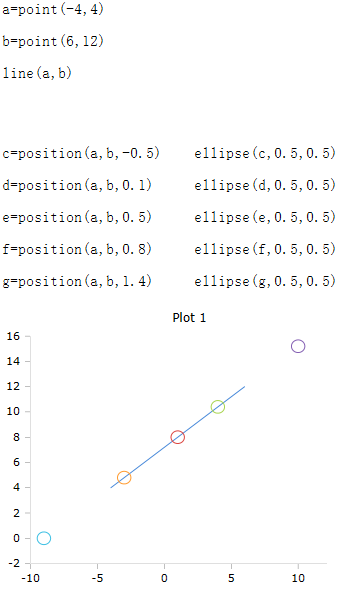# Position Function

Bcalculates the position on the line

### Description

The function $$Position$$ calculates a position on the line of two points in the coordinate system. The result is an object of the class $$Point$$, which contains among other things the x- and y-coordinates of the calculated position.

### Syntax

Position (x1, y1, x2, y2, pos)

Position (Point(x, y), Point(x, y), pos)

### Parameter

As parameters, the X / Y coordinates or two objects of the class Point are passed. The parameter pos determines the position to be calculated.

A value of pos between 0 and 1 determines a position between the two points. The position 0 is identical to the first point.

With a negative value of pos, the calculated position lies on the straight line below point 1. If the value is >1, the calculated position is on the line above point 2.

### Example

The following example calculates the middle between the two points.

The line in the graph shows the straight line between the points. The circle marks the center.The following example calculates 5 positions on the line.